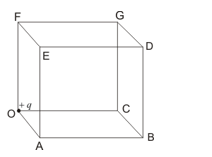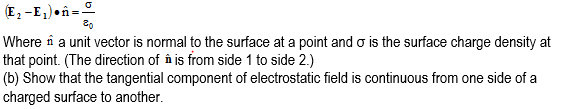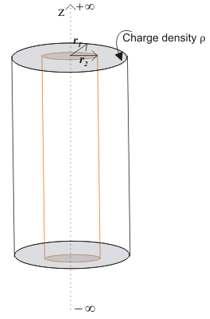Important questions on Gauss's Law for JEE Main and Advanced

Question 1
Consider the figure given belowA positive charge +q is placed at corner of the cube. Find the electric flux through the right face BCGDB of the cube.
Question 2
Consider a sphere of radius r having charge q C distributed uniformly over the sphere. This sphere is now covered with a hollow conducting sphere of radius R>r.
1. Find the electric field at point P away from the centre O of the sphere such that r<OP<R.
2. Find the surface charge density on the outer surface of the hollow sphere if charge q’ C is placed on the hollow sphere.

Question 3
1. Find the electric field inside the uniformly charged sphere of radius R and volume charge density ρ using Gauss’s law.
2. Use Gauss’s law to find the electric field outside, at a point on the surface and at any point inside a spherical shell of radius R, carrying a uniform surface charge density σ.

Question 4
(a) Show that the normal component of electrostatic field has a discontinuity from one side of a charged surface to another given byQuestion 5
Consider a cylinder as given below in the figureVolume between radius r1 and r2 contains uniform charge density ρ C/m3. Use Gauss’s law to find electric field in all regions.

Solutions